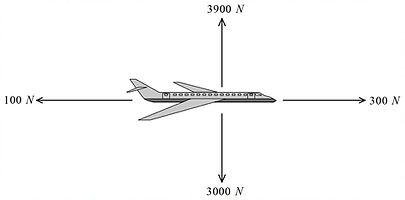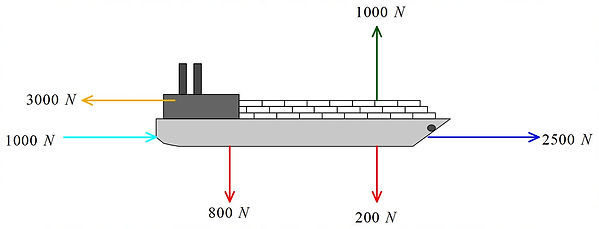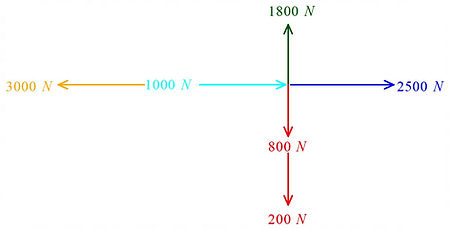## 1.2 Forces

For a still object to be able to move, a force has to be applied. Similarly if that object is to be stopped a force must be applied in a direction opposite to its motion. Therefore, force has both magnitude and direction meaning it is a vector. Usually an object as more than force acting on it in different direction. For us to understand how the object will move because of these forces we have to understand the rules we have to follow when combining them. This chapter will focus on moments, Newton's laws of motion and momentum.

Let's first look at adding forces:

Let's start by looking at an example.Example 1:

Let's assume that we have an airborne airplane. It has two forces of 100 N each propelling it forward. There is an opposing force acting on it due to air resistance. Combine all these forces to calculate a resultant force that's acting on it.

How do we do this? First we are going to have to assume that the forces are acting on a straight line. It other words it is acting only on the x-axis meaning there is no force on the y-axis. Therefore, it this case its just simply adding or substracting the forces together. We will use the right as the positive direction:

+100 N +100 N -30 N = +170 N. We can conclude that the plane is moving forward, not that it could ever fly in reverse.

Okay, so that was very easy because the forces were acting on a straight line. What if the forces were perpendicular to each other? Can we still just add or substract the forces together to get the resultant force. No you can't because they are acting on different axes. Let's look at another example to figure it out:Example 2:

This time let's assume that we have four different forces acting on the plane. One pair along the x-axis and another along the y-axis. By following example 1 we can simplify this by substracting the forces acting along the same axis. By doing so we can simplify it to get the image below:

Now we have a set of forces that are perpendicular to each other. We can simply substract or add them. How do we deal with this to determine the resultant force. As shown in the image below we can rewrite the perpendicular forces side by side. By doing  so we get a right-angled triangle which means we can solve for the resultant force using pythagoras theorem.

Okay so we found out the magnitude of the resultant force. But what about the direction? We know that the direction must be in between north and east. How can we determine the exact direction? We can use trigonometry again. Looking back at the triangle we made, we can see that we know the adjacenst side (200 N) and we know the opposite side (900 N). Using inverse of tan we can caculate the angle:Let's look at another example before moving on:Example 3:

The image below shows a cargo ship with various forces acting on it. The forces are perpendicular.

Let's combine the forces together so that we can add or substract the forces acting along the same plane.

Now that we have a clearer picture we can simplify it to get a pair forces that are perpendicular to each other:Finally we can use pythagoras theorem to determine the resultant force. Then we can use inverse of tan to calculate the angle of the resultant force.

That wasn't soo difficult. But what if the forces were not perpendicular? How would one calculate the resultant force in such a situation?

In such cases you have to try to manipulate the vectors to get a right angled triangle. Let's look at an example:Now that we know what the x and y components of that force is, we can redraw the force diagram. As you can see below, the forces are perpendicular.We can substract the 114.7 N from the 250 N since they are acting along the same plane in opposite directions. Our force diagram will now look like this:We can now use Pythagoras theorem to calculate what our new resultant force is. Then, we can use inverse tan to solve for the angle of the resultant force as shown below:If we put the new resultant force on our diagram we can see that the plane is being pulled in the opposite direction. This means that unless the pilot changes the output of the engine the plane will not be able to maintain its trajectory.Example 4:

A plane is flying with a resultant force of 200 N at an angle of 55 degrees with with respect to the +ve x-axis as shown below. But due to sudden turbulence there is a new force acting on the plane opposite to its motion. The new drag force is 250 N acting along the x-axis of the plane. Will the plane be able to maintain its trajectory at current engine power?

Let's draw a force diagram.

As you can see we can't use Pythagoras to solve this yet. What we can do, is to separate the 200 N force into its x and y components. To get the x component we multiply 200N by cos 55. To get the y component we multiply by sin 55 as shown below:

### Newton's Laws of Motion

Let's do a quick review of the laws of motion. As we know Sir Isaac Newton was a genius. His contributions to physics and mathematics were paramount for the progress of science. Calculus and a lot of the concepts you will learn in physics was invented by him.

First Law

If an object has no external force acting on it or if all forces acting on it balance out to zero, then the object will maintain its original motion. That is to say that if the object was not not moving then it will stay at rest or if it was moving then it would keep moving at that constant velocity. Therefore, the resultant force is zero and the acceleration of the object is also zero.

Second Law

The second law deals with an object that is under a resultant force. For a system with a constant mass, a resultant force will change its motion by causing a change in velocity. We can come up with a mathematical equation to explain this: